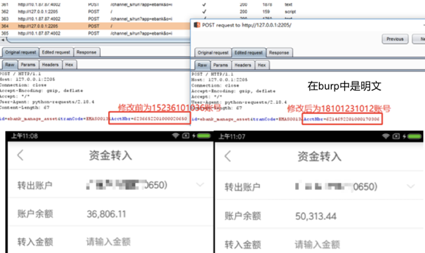## 1.Background

（1）加密算法不复杂，就是AES_CBC_128，但是key和IV的生成算法十分复杂；

（2）key和IV值约10分钟更新一次，更新过程采用RSA加密；

（3）加密逻辑封装在第三方库中，代码经过混淆，这在复杂算法逻辑中起到了较好的防护作用，难以分析清楚；

（4）不能花太多时间在分析逻辑、复现逻辑、编写插件上面。于是就在加解密前进行明文数据处理，解密后进行数据显示。

## 2.LUA & Send Data

GetMethodId(env, claz, “postAsyn”, “(Lcom/xxxxxx/emp/lua/java/CLEntity;Ljava/lang/String;Ljava/lang/String;III)V”)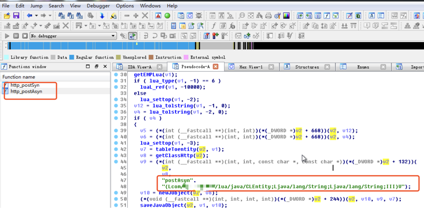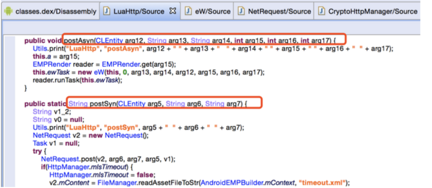NetRequest中的post方法如下，调用了sendPostRequest方法（见图4）。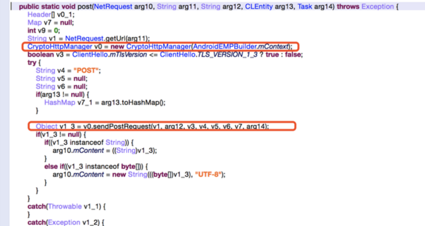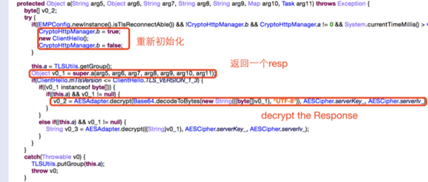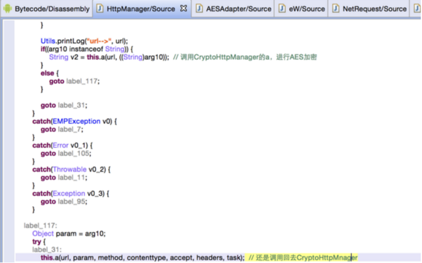## 3.Hook & Modify

```  // 发送的明文数据
var cryptoHttpMgr = Java.use("com.xxxxxx.emp.net.CryptoHttpManager");
// 解密的返回数据
```

```request_method.implementation = function(url, param, rsq_method, contenttype,
send("[+] Requesting .... "); // send将固定字符 以及 数据发送出来

send("- Req Method: " + rsq_method);
send("- Req URL: " + url);
send("- Req Params: " + param.toString());

};

decryptByte.implementation = function(content, key, iv){
send("[+] Decrypting .... ");

send("- AES key:\\n" + hexdump(b2s(key)));
send("- AES IV:\\n" + hexdump(b2s(iv)));

var result = decryptByte.call(this, content, key, iv);

send("- out:\\n" + hexdump(b2s(result)));

return result;
};
```

```def on_message(message, data):
if message['type'] == 'send':
```

```function hexdump(buffer, blockSize) {
blockSize = blockSize || 16;
var lines = [];
var hex = "0123456789ABCDEF";
for (var b = 0; b < buffer.length; b += blockSize) {
var block = buffer.slice(b, Math.min(b + blockSize, buffer.length));
var addr = ("0000" + b.toString(16)).slice(-4);
var codes = block.split('').map(function(ch) {
var code = ch.charCodeAt(0);
return " " + hex[(0xF0 & code) >> 4] + hex[0x0F & code];
}).join("");
codes += "   ".repeat(blockSize - block.length);
var chars = block.replace(/[^\x20-\x7E]/g, '.'); // 不可打印字符
if (chars.charAt(chars.length - 1) == '\\'){ // 打印\这种符号的时候，在Python中直接把后面的字符转义了，所以要修正
chars += '\\';
}
chars += " ".repeat(blockSize - block.length);
lines.push(addr + " " + codes + "  " + chars);
}
return lines.join("\\n");
}
function b2s(array) {
var result = "";
for (var i = 0; i < array.length; i++) {
result += String.fromCharCode(modulus(array[i], 256));
}
return result;
}
function modulus(x, n) {
return ((x % n) + n) % n;
}
```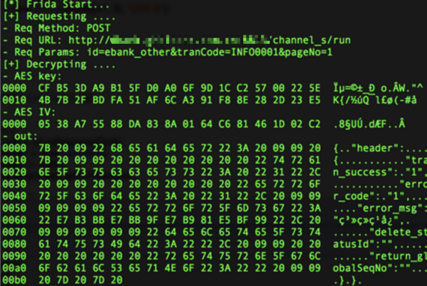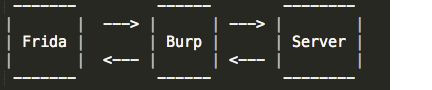```from BaseHTTPServer import HTTPServer, BaseHTTPRequestHandler
from optparse import OptionParser

ECHO_PORT = 2205

class RequestHandler(BaseHTTPRequestHandler):

def do_POST(self):
request_path = self.path

length = int(content_length) if content_length else 0

self.send_response(200)

def main():
print('Listening on localhost:%d' % ECHO_PORT)
server = HTTPServer(('', ECHO_PORT), RequestHandler)
server.serve_forever()

if __name__ == "__main__":
print("[x] Starting echo server on port %d" % ECHO_PORT)
main()
```

• 请求特征 —— id=
• 请求长度 —— 必定是大于14的
• 传输标志 —— Req Params

```def on_message(message, data):
if message['type'] == 'send':
r = requests.post("http://" + BURP_HOST + ":" + str(BURP_PORT), data = payload, proxies = proxies);
if r.status_code == 200:
else:
print(message)
```

```       var op = recv('modify', function onMessage(modMessage) { // 接收回传回来的type为modify的内容
send("- Fix Params: " + modMessage['payload']); // 打印修改后的值
});
op.wait(); // 同步等待
```

## 4.Repeating

（1）发送一个Burp请求。

（2）等待返回。

• 如果有asd（随手写的）-> 进入（3）；
• 如果没有asd -> 跳过循环 -> 调用程序方法（循环跳出的会执行多一次call）。

（3）去除asd标签。

（4）调用程序方法。

（5）加上asd标签，回到1。

```while(1){
send("[*] Repeating .... ");
send("- Req params: " + param); // 1
var op = recv('mod_req', function onMessage(modMessage) { //2
send("- Fix Params: " + modMessage['payload']);
});
op.wait();

if (param.indexOf('asd') < 0) break; // 2.2
//2.1
param = param.replace(/asd/,""); // 3
param = param + 'asd'; // 5
}
```

python中的信息处理代码如下：

```def on_message(message, data):
global theflag
global vericode
if message['type'] == 'send':
typestr = 'mod_req'
r = requests.post("http://" + BURP_HOST + ":" + str(BURP_PORT), data = payload, proxies = proxies);
if r.status_code == 200:
else:
print(message)
```

（1）利用SimpleHTTPServer监听一个端口用于接收Burp的请求。

（2）用异步消息接收被post过来的消息（数据是端口监听的数据）。

（3）接收到数据后进行函数调用即可。

## 5.成果检验